• 这个是用来计算多元线性回归方程的算法，可以代替matlab中的函数，结果绝对正确
• 数据分析基础-广义多元线性回归方程的构建，数据分析基础-广义多元线性回归方程的构建，数据分析基础-广义多元线性回归方程的构建
• ## 多元线性回归方程原理及其推导

万次阅读 多人点赞 2018-10-21 14:19:22
1.在统计学中，线性回归方程是利用最小二乘函数对一个或多个自变量之间关系进行建模的一种回归分析。这种函数是一个或多个称为回归系数的模型参数的线性组合。只有一个自变量的情况称为简单回归，大于一个自变量的...
多元线性方程原理及推导
概念
1.在统计学中，线性回归方程是利用最小二乘函数对一个或多个自变量之间关系进行建模的一种回归分析。这种函数是一个或多个称为回归系数的模型参数的线性组合。只有一个自变量的情况称为简单回归，大于一个自变量的情况叫多元回归。
2.在线性回归中，数据使用线性预测函数来建模，并且未知的函数模型参数也是通过数据来估计。这种模型被叫做线性模型。最常用的线性回归建模是给定的X的条件下y的条件分布的分位数作为X的线性函数表示。像所有形式的回归分析一样，线性回归也是把焦点放在给定X的值的y的条件概率分布，而不是X和y的联合概率分布（多元分析领域）。
公式
一元线性回归方程：y=a+bx
a称为截距
b为回归直线的斜率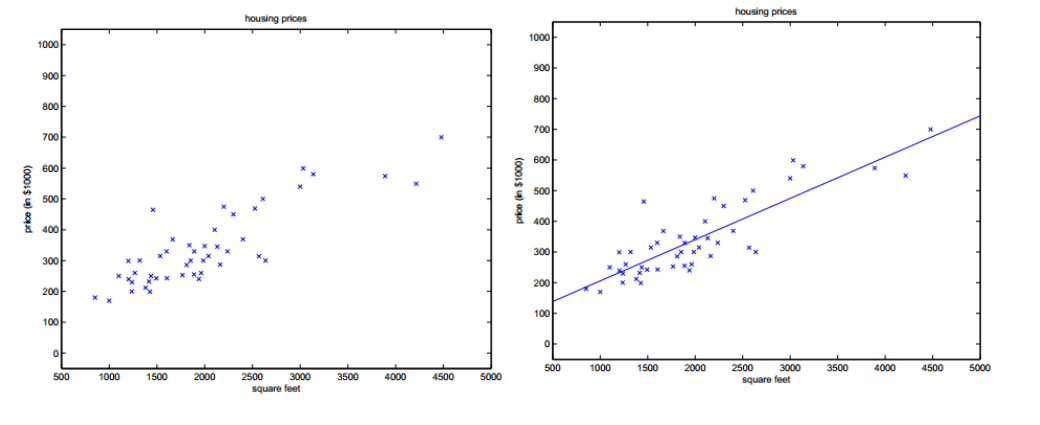多元线性回归方程：y=b0+b1X1+b2X2+…+bnXn
b0为常数项
b1,b2,b3,…bn称为y对应于x1,x2,x3,…xn的偏回归系数
推导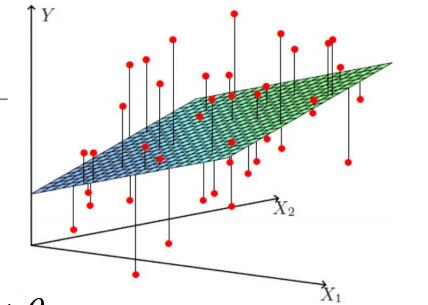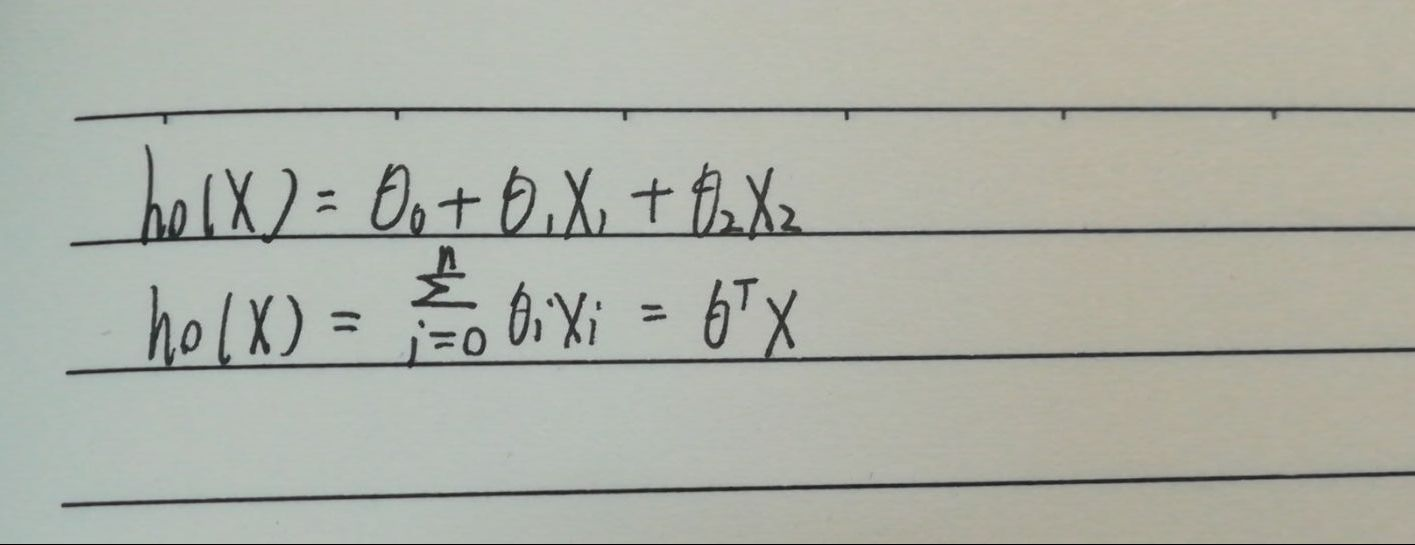使用极大函数解释最小二乘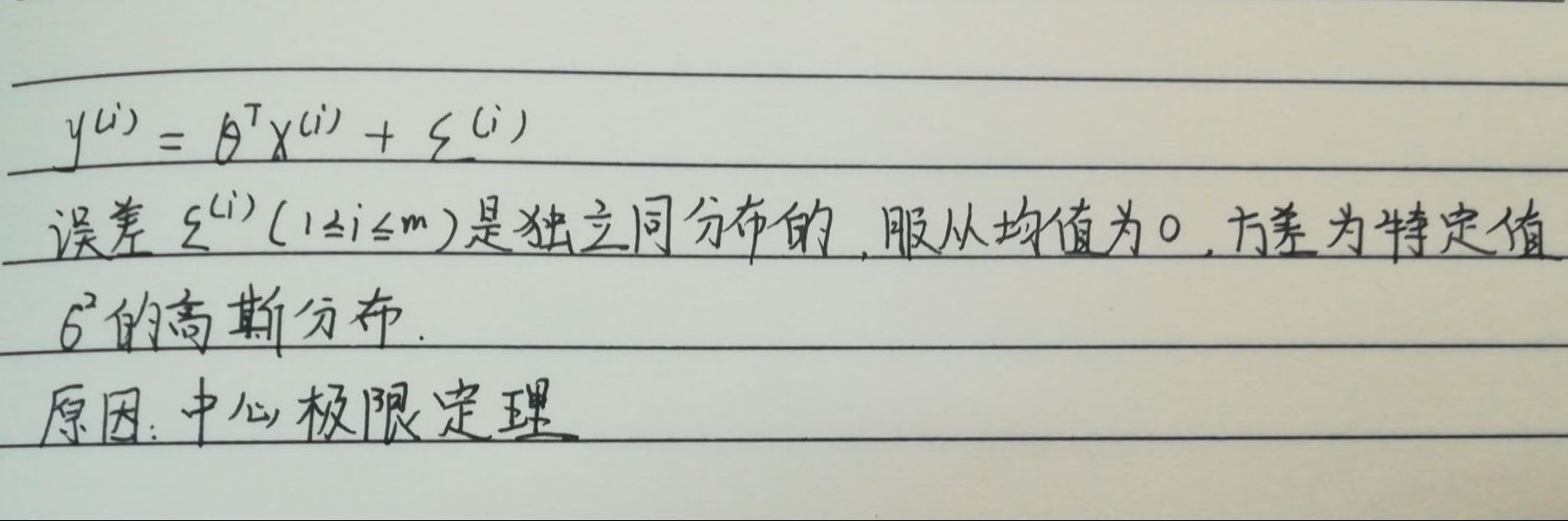似然函数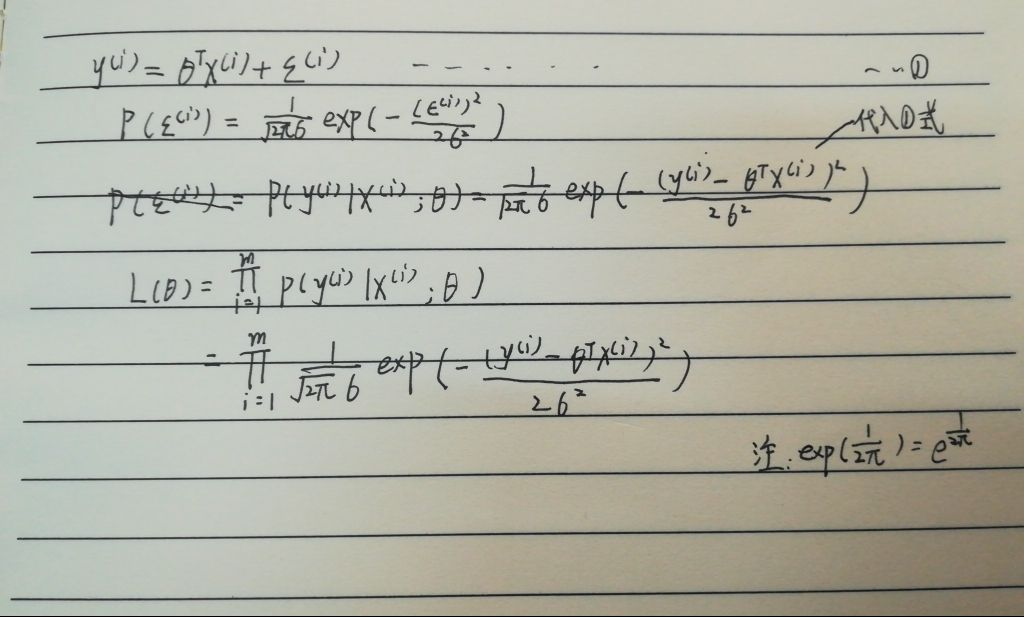高斯的对数似然与最小二乘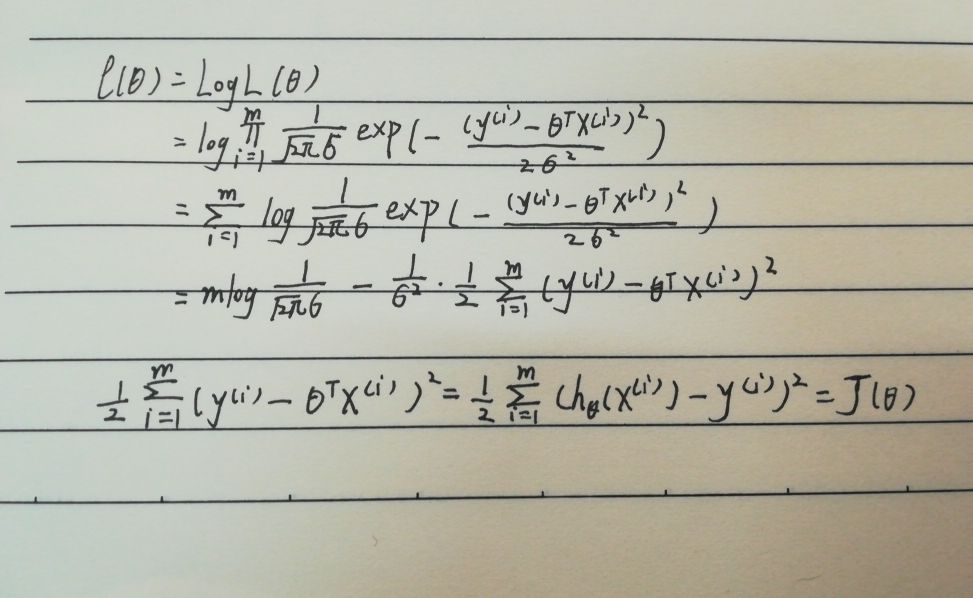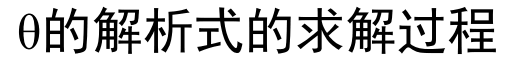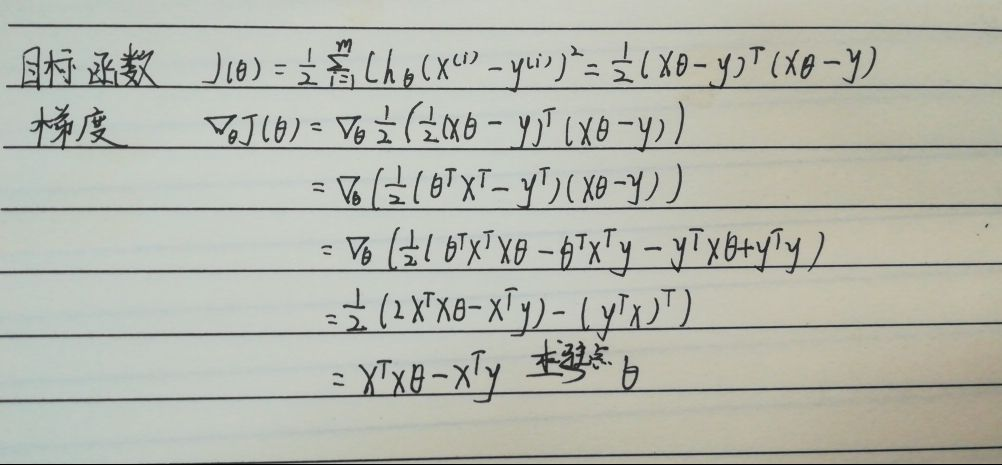参数的解析式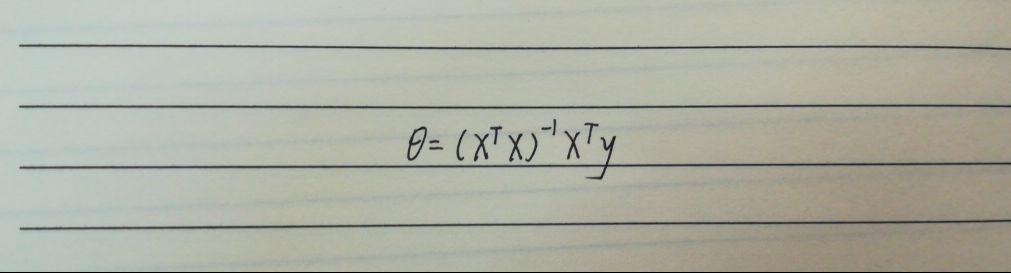总结
    自我认为多元线性方程的推导过程还是挺麻烦的，需要多看几遍。学习推导的过程中，我们要认识一些数学符号，一些方法，比如最小二乘，极大似然，梯度等等。
事实上，一种现象常常是与多个因素相联系的（比如：房子总价与房子面积，房间数，地段这些因素相关），由多个自变量的最优组合共同来预测或估计因变量，比只用一个自变量进行预测或估计更有效，更符合实际。因此多元线性回归比一元线性回归的实用意义更大。



展开全文线性回归
• 多元线性方程组，c++代码编写，包含全部源代码。
• %%1、bint表示回归系数区间估计 %2、r表示残差 %3、rint代表置信区间 ...% r^2越接近于1，回归方程越显著 %alpha表示显著水平 %% x=[143 144 145 147 148 150 153 154 155 156 157 158 159 160 1...
%%1、bint表示回归系数区间估计可参考http://www.360doc.com/content/11/0801/20/2537127_137246007.shtml
%2、r表示残差
%3、rint代表置信区间
%4、stas表示用于检验回归模型的统计量，有三个数值 r^2 F 与F对应的概率P 例如p<0.05 残差95%
%   r^2越接近于1，回归方程越显著
%alpha表示显著水平

%%
x=[143 144 145 147 148 150 153 154 155 156 157 158 159 160 161 162]';
X=[ones(16,1),x];
Y=[87 85 88 91 92 90 93 95 98 98 97 95 97 99 100 102]';
[b,bint,r,rint,stats]=regress(Y,X)
t=1:16;
%%
figure(1);
y_fitting=X(t,:)*b;
plot(t,y_fitting,'r-',  t,Y(t,:),'b-', t,abs(y_fitting-Y(t,:)),'k-');
legend('红--拟合值','蓝--实际值','黑--误差值');
text(3,50,strcat('相关系数R=',num2str(stats(1,1 ))));
text(7,50,strcat('F=',num2str(stats(1,2))));
text(9,50,strcat('P=',num2str(stats(1,3 ))));
nhfcs1=strcat('拟合方程式',num2str(b(1,1)),'+',num2str(b(2,1)),'*X1');
text(11,50,nhfcs1);
%
%功能 在当前轴中创建text对象。函数text是创建text图形句柄的低级函数。可用该函数在图形中指定的位置上显示字符串。

%用法 text(x,y,'string')在图形中指定的位置(x,y)上显示字符串string

%text(x,y,z,'string') 在三维图形空间中的指定位置(x,y,z)上显示字符串string
%
title('线性回归曲线拟合结果');
xlabel('样本点');
ylabel('分数');

%%
figure(2);
ul=rint(:,1);
I1=rint(:,2);
plot(t,I1,'b-', t,r,'R*',  t, ul,'g-');
legend('蓝色--残差95%置信区间上限','红--残差值','绿--残差95%置信区间下限');
xlabel('样本值');
ylabel('残差值');
figure(3)
rcoplot(r,rint);   %残差分析，作残差图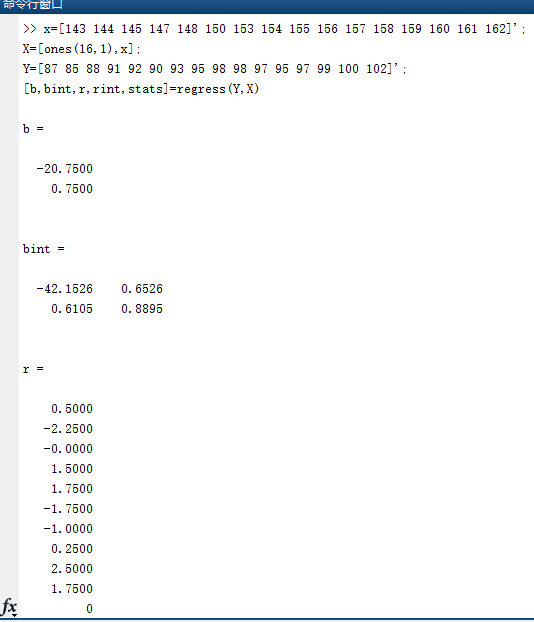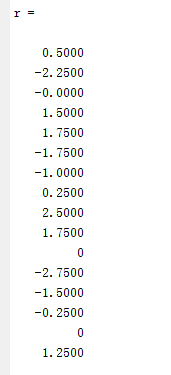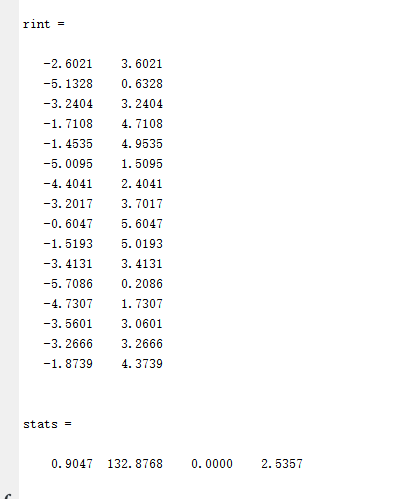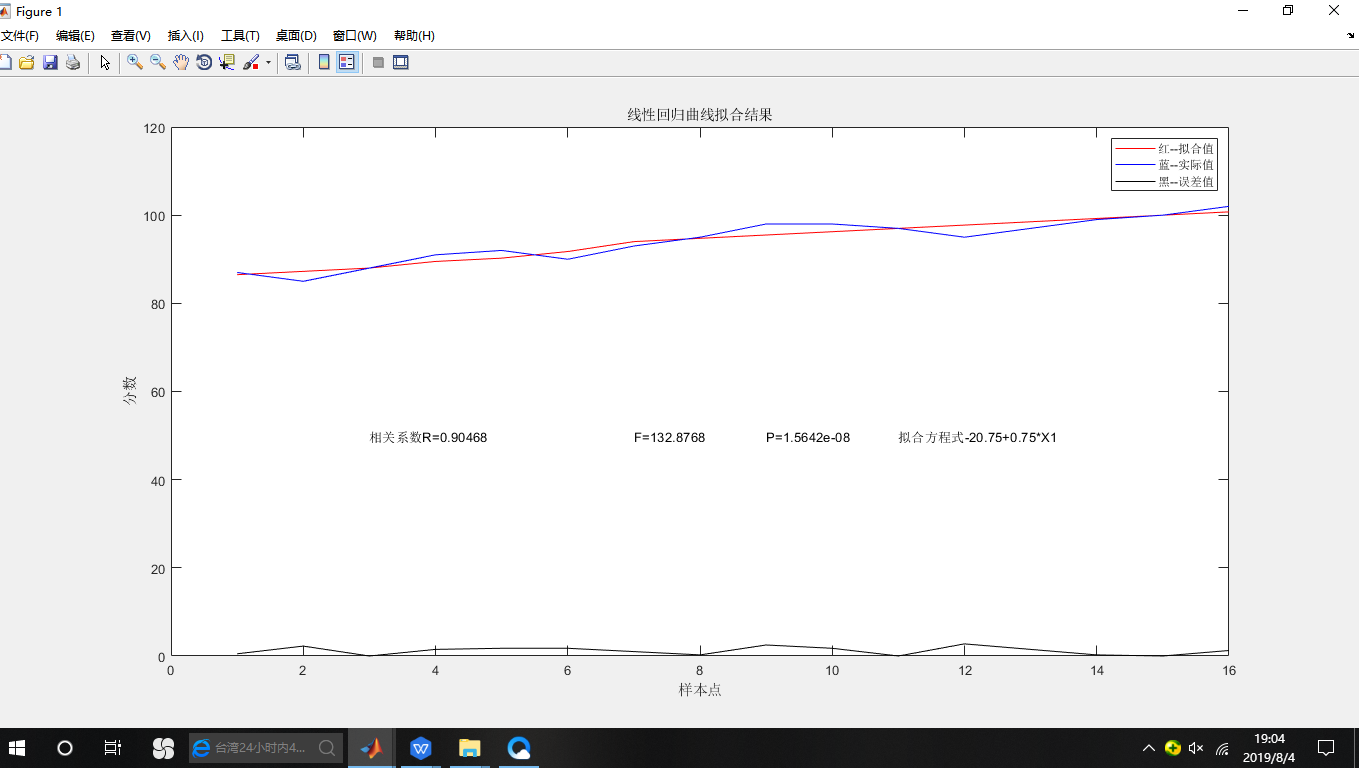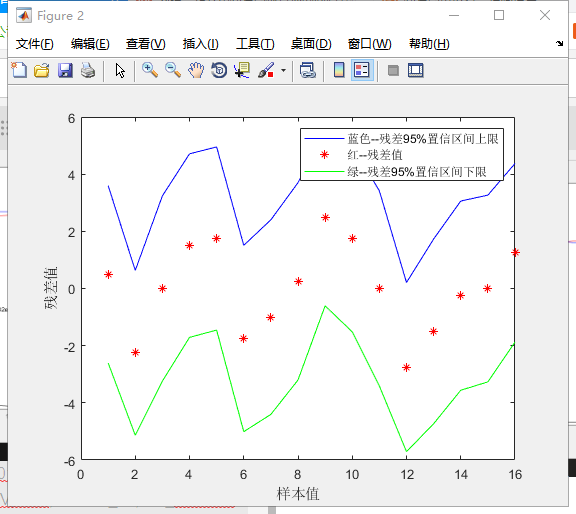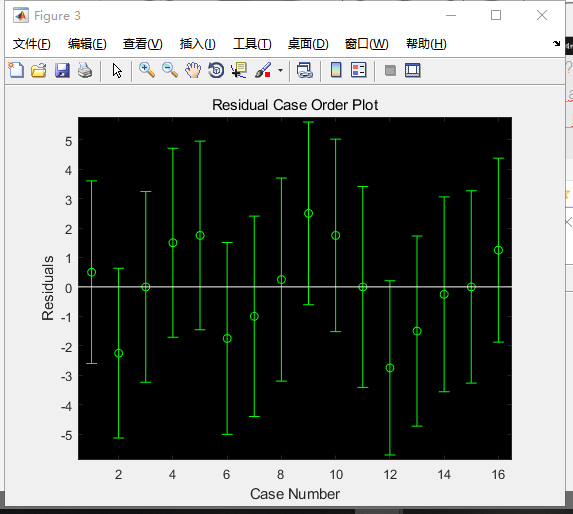残差图(Residual Plots)
我们可以用残差图来估计观察或预测到的误差error(残差residuals)与随机误差(stochastic error)是否一致。用一个丢骰子的例子最好理解了。当你丢出去一个六面的骰子时，你不应该能够预测得到哪面点数向上。然而，你却可以评估在一系列投掷后，正面向上的数字是否遵循一个随机模式，你自己心中就会想象出一个随机散布的残差图。如果，有人背着你对骰子做了点手脚，让六点更频繁的出现向上，这时你心中的残差图看上去就似乎有规律可循，从而不得不修改心中的模型，让你狐疑骰子一定有问题。
相同的原则也适用于回归模型。你不应该能够预测任何给定的观察或预测结果的错误(或者说差别)。你需要确定残差是否与随机误差相互呈现一致性，就像丢骰子一样，残差若整体呈现“很古怪”的模式，你就需要回头修改你的回归模型了。上面“古怪”究竟怎么看呢？看下文。

%clc
%clear
%%
%目标函数：y=Ax1^2+Bx2^2+Cx1+Dx2+Ex1*x2+F  （这是一个二次函数，两个变量，大写的字母是常数）
%导入数据
y=[7613.51  7850.91  8381.86  9142.81 10813.6 8631.43 8124.94 9429.79 10230.81 10163.61 9737.56 8561.06 7781.82 7110.97]';
x1=[7666 7704 8148 8571 8679 7704 6471 5870 5289 3815 3335 2927 2758 2591]';
x2=[16.22 16.85 17.93 17.28 17.23 17 19 18.22 16.3 13.37 11.62 10.36 9.83 9.25]';
X=[ones(size(y)) x1.^2 x2.^2 x1 x2 x1.*x2];
%开始分析
[b,bint,r,rint,stats] = regress(y,X)
scatter3(x1,x2,y,'filled') %scatter可用于画散点图a
hold on
%%
%拟合，三维视图显示
hold on  %不要清除计算数据，在刚刚那副散点图上接着画
x1fit = min(x1):100:max(x1);   %设置x1的数据间隔
x2fit = min(x2):1:max(x2);     %设置x2的数据间隔
[X1FIT,X2FIT] = meshgrid(x1fit,x2fit);  %生成一个二维网格平面，也可以说生成X1FIT,X2FIT的坐标
YFIT=b(1)+b(2)*X1FIT.^2+b(3)*X2FIT.^2+b(4)*X1FIT+b(5)*X2FIT+b(6)*X1FIT.*X2FIT;    %代入已经求得的参数，拟合函数式
mesh(X1FIT,X2FIT,YFIT)    %X1FIT，X2FIT是网格坐标矩阵，YFIT是网格点上的高度矩阵
view(10,10)  %改变角度观看已存在的三维图，第一个10表示方位角，第二个表示俯视角。
%方位角相当于球坐标中的经度，俯视角相当于球坐标中的纬度
xlabel('x1') %设置X轴的名称
ylabel('x2') %设置y轴的名称
zlabel('y')  %设置z轴的名称
hold on

%%
figure(2)
rcoplot(r,rint);   %残差分析，作残差图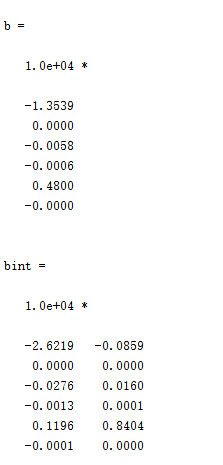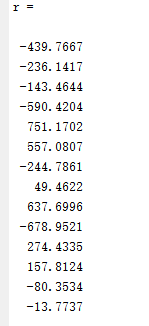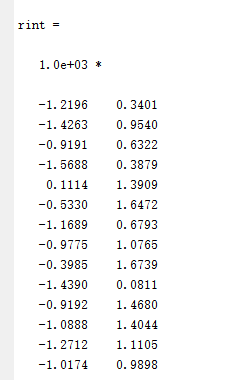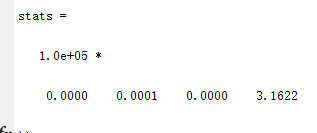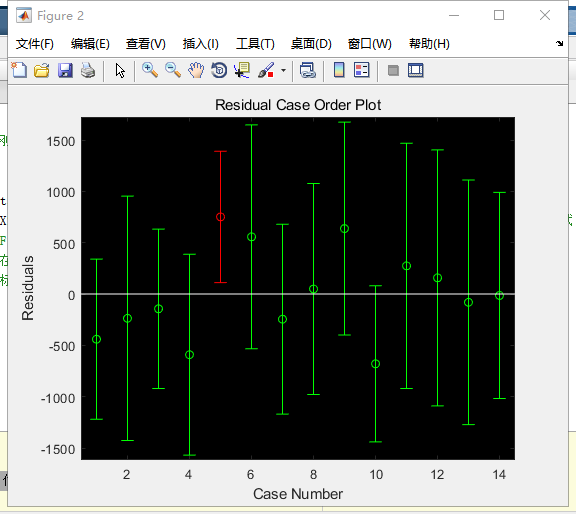https://zhuanlan.zhihu.com/p/20700731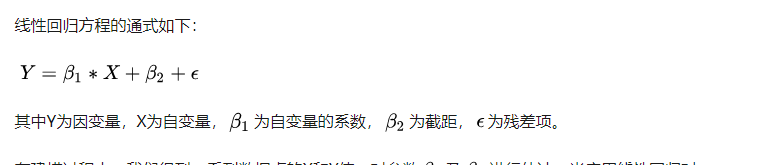2回归值与残差的残差图编辑
为检验建立的多元线性回归模型是否合适，可以通过回归值与残差的散点图来检验。其方法是画出回归值与普通残差的散点图，或者画出回归值与标准残差的散点图，其图形可能会出现下面三种情况(如图1所示)：
图1（a）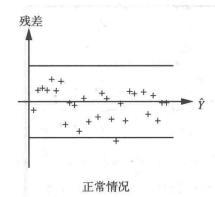图1（b）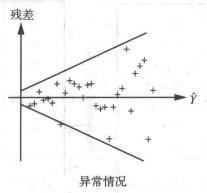对于图1(a)，如果大部分点都落在中间(b)部分，而只有少数几个点落在外边，则这些点对应的样本，可能有异常值存在。
图1（c）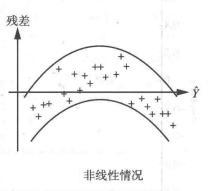展开全文• 通过对某矿11-2煤层瓦斯...结果显示11-2煤层的瓦斯含量主控因素为底板标高,其与主断层距离也是影响瓦斯含量的重要因素,多元线性回归方程预测值与实测值相近,准确性较高。该方法可为煤层瓦斯含量预测提供一种新途径。
• 一、简单的多元线性回归：data.txt1,230.1,37.8,69.2,22.12,44.5,39.3,45.1,10.43,17.2,45.9,69.3,9.34,151.5,41.3,58.5,18.55,180.8,10.8,58.4,12.96,8.7,48.9,75,7.27,57.5,32.8,23.5,11.88,120.2,19.6,11.6,13.29...
一、简单的多元线性回归：data.txt1,230.1,37.8,69.2,22.12,44.5,39.3,45.1,10.43,17.2,45.9,69.3,9.34,151.5,41.3,58.5,18.55,180.8,10.8,58.4,12.96,8.7,48.9,75,7.27,57.5,32.8,23.5,11.88,120.2,19.6,11.6,13.29,8.6,2.1,1,4.810,199.8,2.6,21.2,10.611,66.1,5.8,24.2,8.612,214.7,24,4,17.413,23.8,35.1,65.9,9.214,97.5,7.6,7.2,9.715,204.1,32.9,46,1916,195.4,47.7,52.9,22.417,67.8,36.6,114,12.518,281.4,39.6,55.8,24.419,69.2,20.5,18.3,11.320,147.3,23.9,19.1,14.621,218.4,27.7,53.4,1822,237.4,5.1,23.5,12.523,13.2,15.9,49.6,5.624,228.3,16.9,26.2,15.525,62.3,12.6,18.3,9.726,262.9,3.5,19.5,1227,142.9,29.3,12.6,1528,240.1,16.7,22.9,15.929,248.8,27.1,22.9,18.930,70.6,16,40.8,10.531,292.9,28.3,43.2,21.432,112.9,17.4,38.6,11.933,97.2,1.5,30,9.634,265.6,20,0.3,17.435,95.7,1.4,7.4,9.536,290.7,4.1,8.5,12.837,266.9,43.8,5,25.438,74.7,49.4,45.7,14.739,43.1,26.7,35.1,10.140,228,37.7,32,21.541,202.5,22.3,31.6,16.642,177,33.4,38.7,17.143,293.6,27.7,1.8,20.744,206.9,8.4,26.4,12.945,25.1,25.7,43.3,8.546,175.1,22.5,31.5,14.947,89.7,9.9,35.7,10.648,239.9,41.5,18.5,23.249,227.2,15.8,49.9,14.850,66.9,11.7,36.8,9.751,199.8,3.1,34.6,11.452,100.4,9.6,3.6,10.753,216.4,41.7,39.6,22.654,182.6,46.2,58.7,21.255,262.7,28.8,15.9,20.256,198.9,49.4,60,23.757,7.3,28.1,41.4,5.558,136.2,19.2,16.6,13.259,210.8,49.6,37.7,23.860,210.7,29.5,9.3,18.461,53.5,2,21.4,8.162,261.3,42.7,54.7,24.263,239.3,15.5,27.3,15.764,102.7,29.6,8.4,1465,131.1,42.8,28.9,1866,69,9.3,0.9,9.367,31.5,24.6,2.2,9.568,139.3,14.5,10.2,13.469,237.4,27.5,11,18.970,216.8,43.9,27.2,22.371,199.1,30.6,38.7,18.372,109.8,14.3,31.7,12.473,26.8,33,19.3,8.874,129.4,5.7,31.3,1175,213.4,24.6,13.1,1776,16.9,43.7,89.4,8.777,27.5,1.6,20.7,6.978,120.5,28.5,14.2,14.279,5.4,29.9,9.4,5.380,116,7.7,23.1,1181,76.4,26.7,22.3,11.882,239.8,4.1,36.9,12.383,75.3,20.3,32.5,11.384,68.4,44.5,35.6,13.685,213.5,43,33.8,21.786,193.2,18.4,65.7,15.287,76.3,27.5,16,1288,110.7,40.6,63.2,1689,88.3,25.5,73.4,12.990,109.8,47.8,51.4,16.791,134.3,4.9,9.3,11.292,28.6,1.5,33,7.393,217.7,33.5,59,19.494,250.9,36.5,72.3,22.295,107.4,14,10.9,11.596,163.3,31.6,52.9,16.997,197.6,3.5,5.9,11.798,184.9,21,22,15.599,289.7,42.3,51.2,25.4100,135.2,41.7,45.9,17.2101,222.4,4.3,49.8,11.7102,296.4,36.3,100.9,23.8103,280.2,10.1,21.4,14.8104,187.9,17.2,17.9,14.7105,238.2,34.3,5.3,20.7106,137.9,46.4,59,19.2107,25,11,29.7,7.2108,90.4,0.3,23.2,8.7109,13.1,0.4,25.6,5.3110,255.4,26.9,5.5,19.8111,225.8,8.2,56.5,13.4112,241.7,38,23.2,21.8113,175.7,15.4,2.4,14.1114,209.6,20.6,10.7,15.9115,78.2,46.8,34.5,14.6116,75.1,35,52.7,12.6117,139.2,14.3,25.6,12.2118,76.4,0.8,14.8,9.4119,125.7,36.9,79.2,15.9120,19.4,16,22.3,6.6121,141.3,26.8,46.2,15.5122,18.8,21.7,50.4,7123,224,2.4,15.6,11.6124,123.1,34.6,12.4,15.2125,229.5,32.3,74.2,19.7126,87.2,11.8,25.9,10.6127,7.8,38.9,50.6,6.6128,80.2,0,9.2,8.8129,220.3,49,3.2,24.7130,59.6,12,43.1,9.7131,0.7,39.6,8.7,1.6132,265.2,2.9,43,12.7133,8.4,27.2,2.1,5.7134,219.8,33.5,45.1,19.6135,36.9,38.6,65.6,10.8136,48.3,47,8.5,11.6137,25.6,39,9.3,9.5138,273.7,28.9,59.7,20.8139,43,25.9,20.5,9.6140,184.9,43.9,1.7,20.7141,73.4,17,12.9,10.9142,193.7,35.4,75.6,19.2143,220.5,33.2,37.9,20.1144,104.6,5.7,34.4,10.4145,96.2,14.8,38.9,11.4146,140.3,1.9,9,10.3147,240.1,7.3,8.7,13.2148,243.2,49,44.3,25.4149,38,40.3,11.9,10.9150,44.7,25.8,20.6,10.1151,280.7,13.9,37,16.1152,121,8.4,48.7,11.6153,197.6,23.3,14.2,16.6154,171.3,39.7,37.7,19155,187.8,21.1,9.5,15.6156,4.1,11.6,5.7,3.2157,93.9,43.5,50.5,15.3158,149.8,1.3,24.3,10.1159,11.7,36.9,45.2,7.3160,131.7,18.4,34.6,12.9161,172.5,18.1,30.7,14.4162,85.7,35.8,49.3,13.3163,188.4,18.1,25.6,14.9164,163.5,36.8,7.4,18165,117.2,14.7,5.4,11.9166,234.5,3.4,84.8,11.9167,17.9,37.6,21.6,8168,206.8,5.2,19.4,12.2169,215.4,23.6,57.6,17.1170,284.3,10.6,6.4,15171,50,11.6,18.4,8.4172,164.5,20.9,47.4,14.5173,19.6,20.1,17,7.6174,168.4,7.1,12.8,11.7175,222.4,3.4,13.1,11.5176,276.9,48.9,41.8,27177,248.4,30.2,20.3,20.2178,170.2,7.8,35.2,11.7179,276.7,2.3,23.7,11.8180,165.6,10,17.6,12.6181,156.6,2.6,8.3,10.5182,218.5,5.4,27.4,12.2183,56.2,5.7,29.7,8.7184,287.6,43,71.8,26.2185,253.8,21.3,30,17.6186,205,45.1,19.6,22.6187,139.5,2.1,26.6,10.3188,191.1,28.7,18.2,17.3189,286,13.9,3.7,15.9190,18.7,12.1,23.4,6.7191,39.5,41.1,5.8,10.8192,75.5,10.8,6,9.9193,17.2,4.1,31.6,5.9194,166.8,42,3.6,19.6195,149.7,35.6,6,17.3196,38.2,3.7,13.8,7.6197,94.2,4.9,8.1,9.7198,177,9.3,6.4,12.8199,283.6,42,66.2,25.5200,232.1,8.6,8.7,13.4回归代码：% A=importdata(‘data.txt‘,‘ ‘,200);%????????A.dataa= load(‘data.txt‘);x1=a(:,) ;x2=a(:,) ;x3=a(:,) ;y=a(:,);X=[ones(length(y),1), x1,x2,x3];[b,bint,r,rint,stats]=regress(y,X);b;bint;stats;rcoplot(r,rint)tx=[230.1,37.8,69.2];b2=[b(2),b(3),b(4)];ty=b(1)+b2*tx‘;ty;简单的得到一个变换的公式y=b(1)+b(2)*x1+b(3)*x2+b(3)*x3;二、ridge regression岭回归其实就是在回归前对数据进行预处理，去掉一些偏差数据的影响。1、一般线性回归遇到的问题在处理复杂的数据的回归问题时，普通的线性回归会遇到一些问题，主要表现在：预测精度：这里要处理好这样一对为题，即样本的数量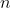和特征的数量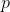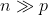时，最小二乘回归会有较小的方差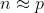时，容易产生过拟合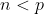时，最小二乘回归得不到有意义的结果模型的解释能力：如果模型中的特征之间有相互关系，这样会增加模型的复杂程度，并且对整个模型的解释能力并没有提高，这时，我们就要进行特征选择。以上的这些问题，主要就是表现在模型的方差和偏差问题上，这样的关系可以通过下图说明：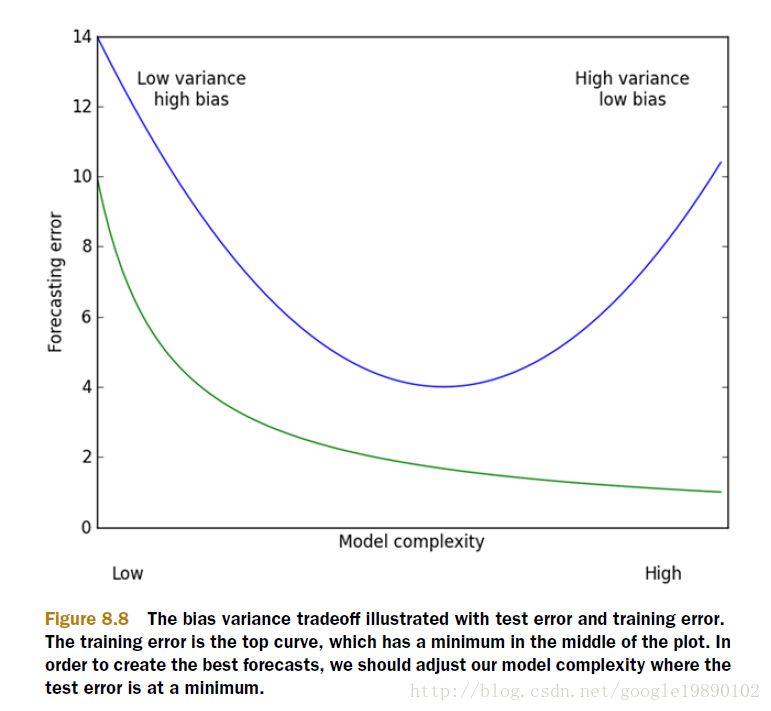(摘自：机器学习实战)方差指的是模型之间的差异，而偏差指的是模型预测值和数据之间的差异。我们需要找到方差和偏差的折中。2、岭回归的概念在进行特征选择时，一般有三种方式：子集选择收缩方式(Shrinkage method)，又称为正则化(Regularization)。主要包括岭回归个lasso回归。维数缩减岭回归(Ridge Regression)是在平方误差的基础上增加正则项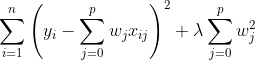,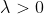通过确定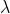的值可以使得在方差和偏差之间达到平衡：随着的增大，模型方差减小而偏差增大。对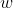求导，结果为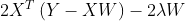令其为0，可求得的值：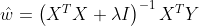3、实验的过程我们去探讨一下取不同的对整个模型的影响。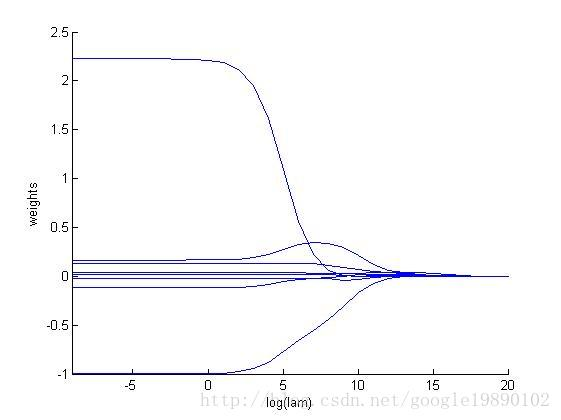MATLAB代码function [ w ] =ridgeRegression( x, y, lam )xTx= x‘*x;[m,n] =size(xTx);temp= xTx + eye(m,n)*lam;if det(temp) == 0disp(‘This matrix is singular, cannot do inverse‘);endw= temp^(-1)*x‘*y;end%% ???(Ridge Regression)clc;%????data= load(‘data.txt‘);[m,n]=size(data);dataX= data(:,2:4);%??dataY= data(:,5);%??%???yMeans=mean(dataY);for i = 1:myMat(i,:)= dataY(i,:)-yMeans;endxMeans=mean(dataX);xVars= var(dataX);for i = 1:mxMat(i,:)= (dataX(i,:) - xMeans)./xVars;end% ??30?testNum= 30;weights= zeros(testNum, n-2);for i = 1:testNumw= ridgeRegression(xMat, yMat, exp(i-10));weights(i,:)= w‘;end% ??????lamhold onaxis([-9 20 -1.0 2.5]);xlabel log(lam);ylabel weights;for i = 1:n-2x= -9:20;y(1,:) = weights(:,i)‘;plot(x,y);endplot出来的图像显示，k=5的时候，出现了拟合，因此取k=5时的w值，% resualt output ,i=5w = ridgeRegression(xMat, yMat, exp(5-10));三、另外一个岭回归比较好的例子function [b,bint,r,rint,stats] =ridge1(Y,X,k)[n,p]=size(X);mx=mean (X);my=mean (Y);stdx=std(X);stdy=std(Y);idx= find(abs(stdx) MX= mx(ones(n,1),:);STDX= stdx(ones(n,1),:);Z= (X - MX) ./ STDX;Y=(Y-my)./stdy;pseudo= sqrt(k*(n-1)) *eye(p);Zplus=[Z;pseudo];Yplus= [Y;zeros(p,1)];[b,bint,r,rint,stats]=regress(Yplus,Zplus);endx=[71.35 22.90 3.76 1158.18 12.20 55.87;67.92 34048 17.11 1494.38 19.82 56.60;79.38 24.91 33.60 691.56 16.17 92.78;87.97 10.18 0.73 923.04 12.15 24.66;59.03 7.71 3.58 696.92 13.50 61.81;55.23 22.94 1.34 1083.84 10.76 49.79;58.30 12.78 5.25 1180.36 9.58 57.02;67.43 9.59 2.92 797.72 16.82 38.29;76.63 15.12 2.55 919.49 17.79 32.07];y=[28.46;27.76;26.02;33.29;40.84;44.50;28.09;46.24; 45.21];x‘*x;count=0;kvec=0.1:0.1:1;for k=0.1:0.1:1count=count+1;[b,bint,r,rint,stats]=ridge1(y,x,k);bb(:,count)=b;stats1(count,:)=stats;endbb‘,stats1plot(kvec‘,bb),xlabel(‘k‘),ylabel(‘b‘,‘FontName‘,‘Symbo l‘)从运行结果及图1可见，k≥0.7时每个变量相应的岭回归系数变化较为稳定，因而可选k=0.7，建立 岭回归方程y=-0.219 5x1-0.120 2x2-0.237 8x3- 0.244 6x4+0.203 6x5-0.249 4x6原文：http://www.cnblogs.com/Anita9002/p/7699550.html
展开全文• 基于jupyter notebook的python编程—–利用梯度下降算法求解多元线性回归方程，并与最小二乘法求解进行精度对比目录一、梯度下降算法的基本原理1、梯度下降算法的基本原理二、题目、表格数据、以及python环境搭建1、...
• jupyter用最小二乘法求解多元线性回归方程 jupyter用最小二乘法求解多元线性回归方程jupyter用最小二乘法求解多元线性回归方程导入需要的数据文件编写程序遇到的问题及解决方法 导入需要的数据文件 首先将所需要的...
jupyter用最小二乘法求解多元线性回归方程
jupyter用最小二乘法求解多元线性回归方程jupyter用最小二乘法求解多元线性回归方程导入需要的数据文件编写程序遇到的问题及解决方法
导入需要的数据文件
首先将所需要的数据文件导入到jupyter中，就可以不用在程序里使用数据文件时加入路径。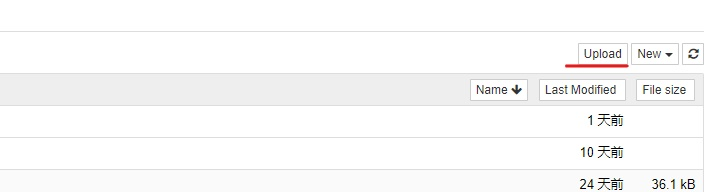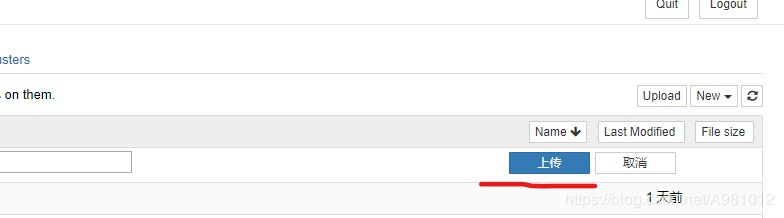编写程序
导入需要的库
import pandas as pd
import numpy as np
import math

然后从表格中读取需要的数据
#准备数据
#如果一个xlsx中有多个表格，就要明确将你所需要的表格的名称填入
x1=p["店铺的面积"]
x2=p["距离最近的车站"]
y=p["月营业额"]

最小二乘法的思想
将自变量、因变量和未知系数写为矩阵，并求得x矩阵的转置矩阵，x转置矩阵与x矩阵相乘之后的逆矩阵，根据公式和提供的自变量和因变量的数据，可以求出未知数矩阵，就可以得到多元线性回归的方程。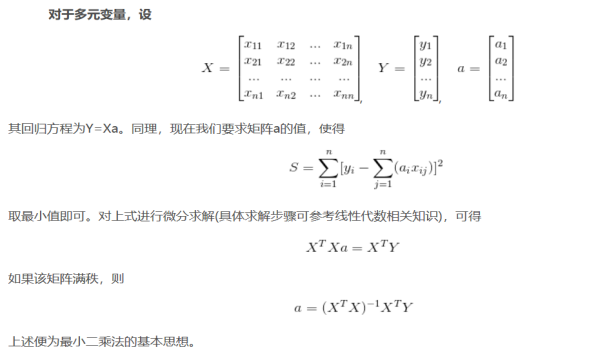n=10#数据个数
h=[]
for i in range(n):
values=[]
values.append(1)
values.append(x1[i])
values.append(x2[i])
h.append(values)
h0=np.array(h)
h1=np.array(h0).T#h1为h的转置矩阵
h2=h1@h0
h3=np.linalg.inv(h2)#求逆矩阵
temp=h3@h1@y

获取未知系数的方式有两种
方式一
temp=np.mat(temp)
w0=temp[0,0]#对应于b
w1=temp[0,1]#对应于a1
w2=temp[0,2]#对应于a2
print("线性回归方程为：y=",w1,"x1",w2,"x2+",w0)

方式二
w0=temp#对应于b
w1=temp#对应于a1
w2=temp#对应于a2
print("线性回归方程为：y=",w1,"x1",w2,"x2+",w0)

已经求得多元线性回归方程，然后就可以根据方程求得判定系数R2
根据漫画统计学之回归分析.pdf，可以得知重回归系数的公式，重回归系数的平方就为判定系数。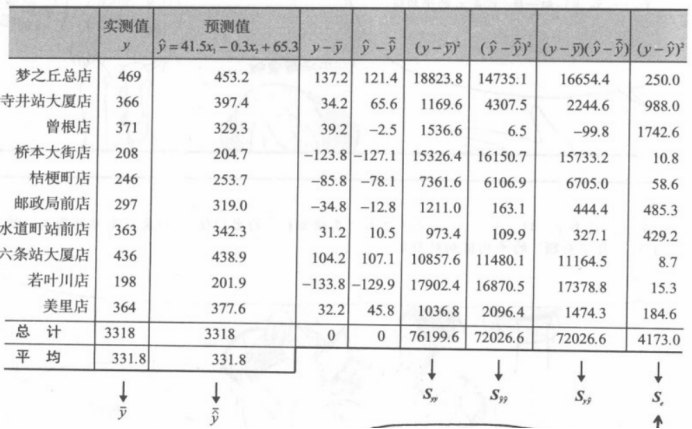根据图片和方程，求得一些需要的数值
y_hat=w1*x1+w2*x2+w0#预测值

先求出y的平均值和预测值的平均值
#求的y的平均值和预测值的平均值
sum1=sum2=0
for i in range(10):
sum1=sum1+y[i]
y1=sum1/n
for i in range(10):
sum2=sum2+y_hat[i]
y_hat1=sum2/n

求y减y的平均值的平方
#求Syy
Syy=0
for i in range(n):
Syy=Syy+((y[i]-y1)*(y[i]-y1))
Syy

求预测值减去预测值的平均值的平方
#求S^y^y
S1y1y=0
for i in range(n):
S1y1y=S1y1y+((y_hat[i]-y_hat1)*(y_hat[i]-y_hat1))
S1y1y

求（y减去y的平均值）*（预测值减去预测值的平均值）
#求Sy^y
S1yy=0
for i in range(n):
S1yy=S1yy+((y[i]-y1)*(y_hat[i]-y_hat1))
S1yy

求y减去预测值的平均值的平方
#求Se
Se=0
for i in range(n):
Se=Se+((y[i]-y_hat[i])*(y[i]-y_hat[i]))
Se

根据这个公式就能求得重回归系数R，进而求得判定系数R2#求R的平方,根据ppt的公式
R=S1yy/math.sqrt(Syy*S1y1y)
R2=R*R
print('R²：',R2)

完整代码：
import pandas as pd
import numpy as np
import math
#准备数据
x1=p["店铺的面积"]
x2=p["距离最近的车站"]
y=p["月营业额"]
n=10#数据个数
h=[]
for i in range(n):
values=[]
values.append(1)
values.append(x1[i])
values.append(x2[i])
h.append(values)
h0=np.array(h)
h1=np.array(h0).T#h1为h的转置矩阵
h2=h1@h0
h3=np.linalg.inv(h2)#求逆矩阵
temp=h3@h1@y
temp=np.mat(temp)
w0=temp[0,0]#对应于b
w1=temp[0,1]#对应于a1
w2=temp[0,2]#对应于a2
print("线性回归方程为：y=",w1,"x1",w2,"x2+",w0)
y_hat=w1*x1+w2*x2+w0#预测值
#求的y的平均值和预测值的平均值
sum1=sum2=0
for i in range(10):
sum1=sum1+y[i]
y1=sum1/n
for i in range(10):
sum2=sum2+y_hat[i]
y_hat1=sum2/n
#求Syy
Syy=0
for i in range(n):
Syy=Syy+((y[i]-y1)*(y[i]-y1))
#求S^y^y
S1y1y=0
for i in range(n):
S1y1y=S1y1y+((y_hat[i]-y_hat1)*(y_hat[i]-y_hat1))
#求Sy^y
S1yy=0
for i in range(n):
S1yy=S1yy+((y[i]-y1)*(y_hat[i]-y_hat1))
#求Se
Se=0
for i in range(n):
Se=Se+((y[i]-y_hat[i])*(y[i]-y_hat[i]))
#求R的平方
R=S1yy/math.sqrt(Syy*S1y1y)
R2=R*R
print('R²：',R2)

遇到的问题及解决方法
在求未知系数w0、w1、w2时，提示出错。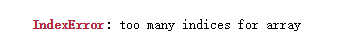添加
temp=np.mat(temp)

将temp[]改为temp[[]]，或者是不添加这句代码，直接将w0=temp[0,0]改为temp
参考博客： https://www.cnblogs.com/LOSKI/p/10639733.html.


展开全文人工智能 python
• jupyter notebook分别用梯度下降和最小二乘法求多元线性回归方程的python编程 在机器学习中，牛顿法是和梯度下降法地位相当的的主要优化算法。 牛顿法 起源:牛顿法以伟大的英国科学家牛顿命名，牛顿不仅是伟大的物理...
• 本文论述多元线性回归的回归系数与偏相关系数之间的数量关系以及他们的统计意义。通过例子说明正确理解这些关系和意义对实际应用的重要性和必要性
• 建立多元线性回归方程，实际上是对多元线性模型（2-2-4）进行估计，寻求估计式（2-2-3）的过程。与一元线性回归分析相同，其基本思想是根据最小二乘原理，求解 使全部观测值 与回归值 的残差平方和达到最小值。...
• 通过sas软件，拟合出多元线性回归方程，接着求出其残差、学生化残差、杠杆量等，进而求出学生化残差，画出QQ图，画出残差图，最后进行BOX-COX变换。
• 前言：本文是作者学习机器学习多元线性回归部分，就消除多重共线性、选择变量问题而做的练习。参照了《R-modeling》.薛毅.P331 例6.10例子，使用到R语言的MASS包，ridge包，lars包。机器学习 LASSO 变量选择
• https://wenku.baidu.com/view/7af0b436f08583d049649b6648d7c1c708a10bbf.html 转载于:https://www.cnblogs.com/fengff/p/9802713.html
• 以上式子被称为多元线性回归的正规方程解（Normal Equation）。多元线性回归可以直接通过数学表达式计算出模型的参数值。 （4）参数意义 2.正规方程解 （1）公式 （2）优点和缺点 缺点：时间...
• 原来就是求三元线性回归的残差啊，害，这有什么难的，妹妹就是不会算权重，一直在网上寻找已经算好权重的数据，为此特意开通了什么会员，咱也不知道咱也不敢问。于是乎，利用自己所学的python，写下了这个程序。 ...python
• 用matlab对多元还原概率预测代码线性回归模型解释 这是一个包含使用Sklearn、pandas、Numpy 和 Seaborn进行线性回归的解释的存储库。 还执行探索性数据分析（EDA）和可视化。 本说明分为以下部分，我们将详细介绍每...
• 控制台应用程序，C++实现多元线性回归，可指定任意几元，根据给定的数据矩阵，训练给出回归方程
• 因此回归方程根据测量值预测测试结果。 A 中的一列提供了回归方程中的常数项。 形式上，我们计算 x 以使平方误差总和 Ax-b 最小化。 较小的平方和意味着 Ax 是 b 的良好预测器。 反斜杠运算符 \（如 x=A\b）或函数...matlab
• ## Python实现多元线性回归

万次阅读 多人点赞 2018-04-12 21:39:25
Python实现多元线性回归 线性回归介绍 线性回归是利用数理统计中回归分析，来确定两种或两种以上变量间相互依赖的定量关系的一种统计分析方法，运用十分广泛。其表达形式为y = w'x+e，e为误差服从均值为0的...Python 数据分析 数据挖掘 pandas
• 背景： 通常样本有多个特征值 选择如下公式是因为它是线性可导的，另外一方面，将限制最大的误差尽可能的小 在这里插入图片描述 多元线性回归方程的正规方程解 ...
• 利用梯度下降算法求解多元线性回归方程，并与最小二乘法求解进行精度对比 基于jupyter notebook的python编程 这里写目录标题利用梯度下降算法求解多元线性回归方程，并与最小二乘法求解进行精度对比基于jupyter ...机器学习
• error(theta0,theta1,theta2,x_data,y_data))) print("多元线性回归方程为:y=",theta1,"X1+",theta2,"X2+",theta0) #画图 ax=plt.figure().add_subplot(111,projection='3d') ax.scatter(x_data[:,0],x_data[:,1],y_...python 算法 深度学习 机器学习...